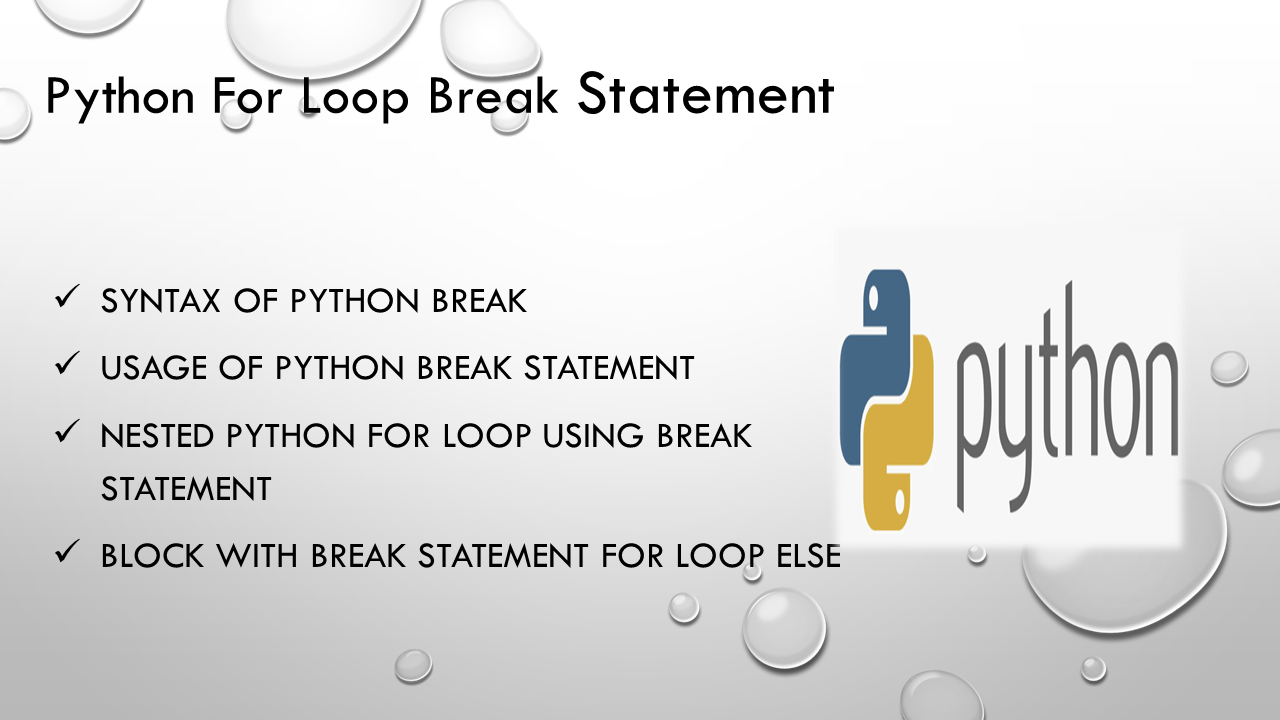# Python For Loop Break Statement

Python `break` statement is used to exit from the for/while loops in Python. For loop iterates blocks of code until the condition is `False`. Sometimes you need to exit a loop completely or when you want to skip a current part of the python for loop and go for the next execution without exiting from the loop. Python allows `break` statement to overcome such situations and you can be well-controlled over your loops. We can also use the break statement in the nested loop to exit the innermost loop.

In this article, I will explain the concept of break statement in Python, and when we apply break statement in a for loop, how it controls the for loops with examples.

## 1. Quick Examples of Python Break Statement

Following are quick examples of a break statement.

``````
# Below are the quick examples

# Example 1: Exit the loop using break statement
courses=["java","python","pandas","sparks"]
for x in courses:
print(x)
if x == 'pandas':
break

# Example 2: placed the print() after the break
courses=["java","python","pandas","sparks"]
for x in courses:
if x == 'pandas':
break
print(x)

# Example 3: Using break statement inside the nested loop
ourses1=["java","python"]
courses2=["pandas","java","python"]
for x in courses1:
for y in courses2:
if x==y:
break
print(x ,y)

# Example 4: Using else block with break statement
courses=["java","python","pandas","sparks"]
for x in courses:
print(x)
if x == 'pandas':
break
else:
``````

## 2. Syntax of Python Break

Following is the syntax of the break statement.

## 3. Usage of Python Break Statement

Sometimes you would like to exit from the python `for`/`while` loop when you meet certain conditions, using the `break` statement you can exit the loop when certain condition meets. The example is given below.

With the `break` statement, you will early exit from the loop.

``````
# Exit the loop using break statement
courses=["java","python","pandas","sparks"]
for x in courses:
print(x)
if x == 'pandas':
break
``````

In the above example, I have taken the `courses` variable as a list which is iterated using for loop. We have applied a `break` statement based on `x == 'pandas'` condition. When the iteration reaches the `pandas` value, the for loop will exit using a `break` statement.

The above example exits for loop when `x` value equals to `pandas`.

Let’s place the print() function after the `if statement`.

``````
# placed the print() after the break
courses=["java","python","pandas","sparks"]
for x in courses:
if x == 'pandas':
break
print(x)
``````

Yields below output

``````
# Output:
java
python
``````

Notice that for loop exited after reaching `x` value ‘`pandas`‘.

## 4. Nested Python For Loop Using break Statement

If a loop presents inside the body of another loop is called a `nested loop`. The inner loop will be executed n number of times for each iteration of the outer loop. The example is given below.

If the `break` statement is inside a nested loop, the `break` statement will end the innermost loop and the outer loop continue executing.

let’s take an example for better understanding.

``````
# Using break statement inside the nested loop
ourses1=["java","python"]
courses2=["pandas","java","python"]
for x in courses1:
for y in courses2:
if x==y:
break
print(x ,y)
``````

In the above example, the inner loop will be executed three times(‘pandas’,’java’,’python’) for each iteration of the outer loop.

Possibilities of the outer loop for the first iteration:

• `java, pandas`
• `java, java` in this case, the condition satisfies so the innermost loop ends. Then the control goes to the outer loop.

Possibilities of the outer loop for the second iteration :

• `python, pandas`
• `python, java`
• `python, python` in this case, the condition satisfies so the innermost loop ends.

Yields below output.

``````
java pandas
python pandas
python java
``````

## 5. For Loop else Block With break Statement

Python allows the `else` keyword with `for` loop. The else block is optional and should be after the body of the loop. The statements in the else block will execute after completing all the iterations of the loop. The program exits the loop only after the else block is executed.

As you learned above, you can use the break statement to exit the loop. In such cases, the else part will not be executed.

``````
# Using else block with break statement
courses=["java","python","pandas","sparks"]
for x in courses:
print(x)
if x == 'pandas':
break
else:
``````

Yields below output

``````
java
python
pandas
``````

## 6. Conclusion

In this article, I have explained the concept of `break` statement in Python, and when we apply break statement in a for loop how it controls the for loops with examples.

Happy learning!!

## Related Articles

### Vijetha

Vijetha is an experienced technical writer with a strong command of various programming languages. She has had the opportunity to work extensively with a diverse range of technologies, including Python, Pandas, NumPy, and R. Throughout her career, Vijetha has consistently exhibited a remarkable ability to comprehend intricate technical details and adeptly translate them into accessible and understandable materials.